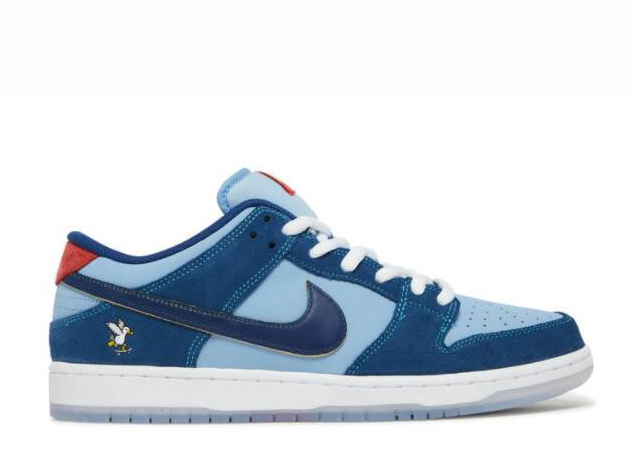All Categories
US\$

#### Cart

Home>Nike>Nike Dunk>Why So Sad X Dunk Low SB The Predatory Bird

# Why So Sad X Dunk Low SB The Predatory Bird

US\$ 108.00
Quality Control:
QC by myself (1-3 days prepation time) QC by FKZ team (Get shipped faster)
Men and GS Sizes:
US4=UK3.5=EUR36=23CM
US\$ 108.00
US4.5=UK4=EUR36.5=23.5CM
US\$ 108.00
US5=UK4.5=EUR37=23.8CM
US\$ 108.00
US5.5=UK5=EUR38=24CM
US\$ 108.00
US6=UK5.5=EUR38.5=24.3CM
US\$ 108.00
US6.5=UK6=EUR39=24.5CM
US\$ 108.00
US7=UK6.5=EUR40=25CM
US\$ 108.00
US7.5=UK7=EUR40.5=25.5CM
US\$ 108.00
US8=UK7.5=EUR41=26CM
US\$ 108.00
US8.5=UK8=EUR42=26.5CM
US\$ 108.00
US9=UK8.5=EUR42.5=27CM
US\$ 108.00
US9.5=UK8.5=EUR43=27.5CM
US\$ 108.00
US10=UK9.5=EUR44=28CM
US\$ 108.00
US10.5=UK10=EUR44.5=28.5CM
US\$ 108.00
US11=UK10.5=EUR45=29CM
US\$ 108.00
US11.5=UK115=EUR45.5=29.5CM
US\$ 108.00
US12=UK11.5=EUR46=30CM
US\$ 108.00
US13=UK12.5=EUR47.5=31CM
US\$ 108.00
Quantity: 0 , Subtotal 0
• Detail
• Product Name: Why So Sad X Dunk Low SB The Predatory Bird
• Item NO.: dx5549 400
• Weight: 0 kg = 0.0000 lb = 0.0000 oz
• Category: Nike > Nike Dunk
• Creation Time: 2023-01-06